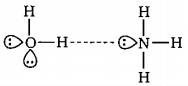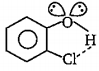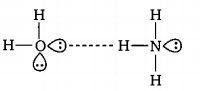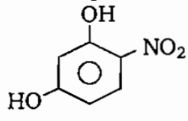In terms of polar character which of the following order is correct ?

(a) $N{H}_{3}<{H}_{2}O    (b) ${H}_{2}S

(c) ${H}_{2}O    (d) $HF<{H}_{2}O

Concept Questions :-

Polarity
High Yielding Test Series + Question Bank - NEET 2020

Difficulty Level:

The correct order or boiling point is:

(a) ${\mathrm{T}}_{2}<{\mathrm{D}}_{2}<{\mathrm{H}}_{2}$

(b) n-pentane<neo pentane

(c) Xe<Ar<He

(d) m-nitrophenol>o-nitrophenol

Concept Questions :-

Types of Bonding
High Yielding Test Series + Question Bank - NEET 2020

Difficulty Level:

The correct order of 'S$—$O' bond length is:

(a) $S{{O}_{3}}^{2-}>S{{O}_{4}}^{2-}>S{O}_{2}>S{O}_{2}$     (b) $S{{O}_{3}}^{2}>S{{O}^{2}}_{4}>S{O}_{2}>S{O}_{3}$

(c) $S{{O}_{4}}^{3}>S{{O}_{3}}^{2}>S{O}_{2}>S{O}_{3}$    (c) $S{{O}_{4}}^{2}>S{{O}_{3}}^{2}>S{O}_{3}>S{O}_{2}$

Concept Questions :-

M.O.T
High Yielding Test Series + Question Bank - NEET 2020

Difficulty Level:

The linear structure is possessed by :

(a) $SnC{l}_{2}$

(b) $NC{O}^{-}$

(c) $N{{O}_{2}}^{+}$

(d) $C{S}_{{}^{2}}$

Concept Questions :-

Hybridisation
High Yielding Test Series + Question Bank - NEET 2020

Difficulty Level:

Cis-butene dioic acid $\stackrel{{\mathrm{K}}_{{\mathrm{a}}_{1}}\left\{-{\mathrm{H}}^{+}\right\}}{⇌}{{\mathrm{X}}^{-}}_{1}\stackrel{{\mathrm{K}}_{{\mathrm{a}}_{2}}\left\{-{\mathrm{H}}^{+}\right\}}{⇌}{\mathrm{X}}_{2}^{2-}$

Trans-butene dioic acid $\stackrel{{\mathrm{K}}_{{\mathrm{a}}_{1}}\text{'}\left\{-{\mathrm{H}}^{+}\right\}}{⇌}{{\mathrm{Y}}^{-}}_{1}\stackrel{{\mathrm{K}}_{{\mathrm{a}}_{2}}·\left\{-{\mathrm{H}}^{+}\right\}}{⇌}{\mathrm{Y}}_{2}^{2-}$

The incorrect statement regarding above the information is:

(a) ${\mathrm{X}}_{2}^{2-}$ species is more basic than ${\mathrm{Y}}_{2}^{2-}$ species

(b) ${\mathrm{X}}_{1}^{-}$ species is more basic than ${\mathrm{Y}}_{1}^{-}$ species

(c) ${\mathrm{K}}_{\mathrm{a}1}$ is greater than $\mathrm{K}{\text{'}}_{\mathrm{a}2}$

(d) $\mathrm{K}{\text{'}}_{\mathrm{a}2}$ is greater than $\mathrm{K}{\text{'}}_{\mathrm{a}2}$

Concept Questions :-

Acid, Base and Fajan's Rule
High Yielding Test Series + Question Bank - NEET 2020

Difficulty Level:

In which of the following compounds octet is complete and incomplete for all atoms:

(a)                  IC               IC             IC                     C                         C

(b)                  C                IC             IC                     C                         IC

(c)                  C                IC             C                      IC                        IC

(d)                 IC                C              IC                    IC                        IC

where C denotes Complete and IC incomplete.

Concept Questions :-

Covalent Bonding
High Yielding Test Series + Question Bank - NEET 2020

Difficulty Level:

In which of the following metal of metal bond is present?

(a) Cupric chloride            (b) stannous chloride

(c) mercurous chloride      (d) mercuric chloride

Concept Questions :-

Types of Bonding
High Yielding Test Series + Question Bank - NEET 2020

Difficulty Level:

Which of the following is not best representation of the H-bond?

(a)(b)(c)(d) None of these

Concept Questions :-

Hydrogen Bonding
High Yielding Test Series + Question Bank - NEET 2020

Difficulty Level:

The pair of elemnts which on combination are most likely to form an ionic compound is :

(a) Na and Ca

(b) K and ${O}_{2}$

(c) ${O}_{2}$ and $C{l}_{2}$

(d)

Concept Questions :-

Ionic Bonding
High Yielding Test Series + Question Bank - NEET 2020

Difficulty Level:

The type of molecular forces of attraction present in the following compound is:(a) Intermolecular H-bonding                   (b) Intramolecular H-bonding

(c) van der Waal's force                           (d) All of these

Concept Questions :-

Hydrogen Bonding